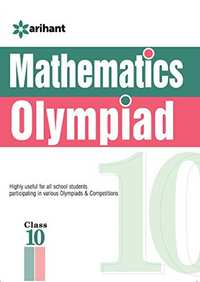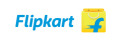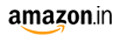Share

# Olympiad Books Practice Sets - Mathematics class 10th CBSE Class 10

SubjectOlympiad Books Practice Sets - Mathematics class 10thBy Arihant Experts (Author)
M.R.P 125/-89.00(29%)97.00(22%)
Product Description

Various institutes and associations across the country conduct Mathematics Olympiads & Competitions for Class 10 students. This specialized book has been designed to provide relevant and the best study material for the preparation for Class 10 students preparing for Mathematics Olympiads like INMO, Regional Mathematical Olympiad (RMO) and other Mathematics Olympiads.
This book has been designed to give the students an insight and proficiency into almost all the areas of mathematics asked in various Mathematics Olympiads. The present book has been divided into 12 chapters namely Real Number, Polynomials, Pair of Linear Equations in Two Variables, Quadratic Equation, Arithmetic Progression, Similarity of Triangles, Coordinate Geometry, Trigonometry, Area Related to Circles, Circles, Statistics and Probability. The book contains complete theory exactly on the pattern of various Mathematics Olympiads with sufficient number of solved examples set according to the pattern and level of Indian National Mathematics Olympiads. Exercises have also been given in the book. Problems from recently held Olympiads have also been given in the book. The book also contains five practice sets designed on the lines of the questions asked in the precious years’ mathematics Olympiads questions. Also answers to solutions for the practice sets have been provided at the end.
As the book contains ample study as well as practice material, it for sure will help aspirants score high in the upcoming Mathematics Olympiads.

S